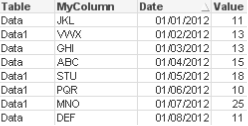# Qlik Design Blog

All about product and Qlik solutions: scripting, data modeling, visual design, extensions, best practices, etc.Employee

## The Concat Function

QlikView has many useful and sometimes underused functions that can make your life as a developer a lot easier. One of these is CONCAT(). This should not be confused with the CONCATENATE script keyword.

In its simplest form, CONCAT() is used to string together values/words/selections in to one string. However, it can be utilized in a number of ways to help you solve different problems.

All of the examples in this post are based on the data in the table below.....I have shown a few simple but useful examples below to hopefully help you in your everyday QlikView development!

Simple String Concatenation

As mentioned earlier, the function lets you string together a list of values. These values can be hard coded or driven my selections/data.

=CONCAT(MyColumn,',')

=CONCAT(DISTINCT MyColumn,',')

This simple concat statement would string together all of the possible values from the column MyColumn. You may wish to add the DISTINCT keyword. This would ensure that each value is only displayed once in the string.

ABC,DEF,GHI,JKL,MNO,PQR,STU,VWX

When using a simple concat, you have the option to add a sort weight to the function to order the string values by a column of your choice. In the example below, I have added the date column to sort the values…..

=CONCAT(MyColumn, ',', Date)

Result: JKL,VWX,GHI,ABC,STU,PQR,MNO,DEF

Concat() within an expression/set statement

There are occasions when you want to pass a selection of values to a set statement. To do this I would need to add some single quotes to the string so that the CONCAT() function returns e.g. 'JKL','VWX'. But you cannot have the single quotes as they are, since they then would be interpreted when the Concat is evaluated instead of when the set expression is evaluated. Instead I use the Chr() function:

=CONCAT(Chr(39)&MyColumn&Chr(39),',')

I can  then  pass this concat statement to the inside of an expression…

=Sum({<MyColumn={\$(=CONCAT(Chr(39)&MyColumn&Chr(39),','))}>} Value)

In most instances, this technique would be used where data islands are present. It lets me pass values to an expression that will not affect any part of the data model as the data island table is not joined to it.

Concat() in the Script

Concat can also be utilised in the script to let you convert multiple rows in to one single column value, just as any other aggregation.

Remembering the source data we have earlier in the blog. The result of script side CONCAT can be seen below...Using Rank() to influence the Concat()

When you start to utilise other function in conjunction with concat() you can start to achieve to clever results. In this example, I have used Rank() to grab the top 3 performers (based on Value) and string them together.

=CONCAT(IF(aggr(Rank(sum(Value)),MyColumn)<=3,MyColumn),',')

Result: ABC,MNO,STU

So as you can see, there are a number of uses for CONCAT(). Let's if you can find more!